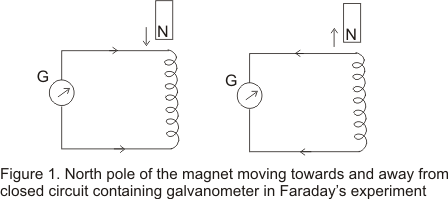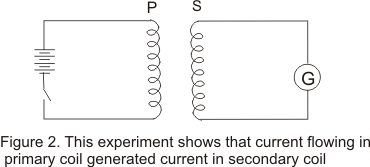# ElectroMagnetic induction

## (1) Introduction

• While studying magnetism we learned that electricty and magnetism are interrelated and infact moving charges or electric currents produces magnetic field that can deflect magnetic compass needle
• A question arises,can moving magnetic field produce electricty .Answer is yes and it was first showed by a british scientist Michael faraday in 1931 who after performing various experiments found that moving magnetic field can give rise to the EMF
• Independently the effect was discovered by Joseph Henry in USA at about the same time
• In this chapter we will discuss about the electric and magnetic field changing with time
• More precisely we will consider the phenomenon related to time changing current or time changing magnetic fields

• faraday in 1831 first discovered that whenever the number it of magnetic lines of forces in a circuit changes ,a emf is produced in the circuit and is known as induced emf and this phenomenon is known as Electro Magnetic Induction
• If the circuit is closed one then a current flows through it which is known an induced current
• This induced emf and current lasts only for the time while magnetic flux is chnaging
• We now illustrate two examples of the sort that faraday and henry performed

(i) experiment I
• Figure below shows a closed circuit containing coil of insulated wire.
• Also note that circuit does not contain any source of emf so there is no deflection in the galvanometer• If we move bar magnet towards the coil keeping the coil stationary with north pole of the magnet facing the coil (say) then we notice deflection in needle of the galvanometer indicating the pressence of the current in the circuit
• This deflection observed is only for the time interval during which the magnet is in motion
• Now if we begin to move the magnet in the opposite direction then the galvanometer needle is now deflected in the opposite direction
• again if we move the magnet towards the coil ,with its south pole facing the coil,the deflection is now in opposite direction,again indicating that the current now setup in the coil is in reverse direction to that when the north pole faces the wire
• A deflection is also oberved in galvanometer when the magnet is held stationary and circuit is moved away from the magnet.
• It is further observed that faster is the motion of magnet ,larger is the deflection in the galavanometer needle.
• From this experiment faraday convinced that magnet moving towards the coil one way has the same effect moving coil towards the magnet the other way.
(ii) experiment -2
• Figure-2 given below shows a primary coil P connected to the battery and a secondary coild connected to the galvanometer• Now we have replaced magnet of the previous experiment with a current carrying coil and expect to observe similar effect as current carrying coil produces magnetic field.
• The motion of either of the coils shows deflection in the galvanometer.
• Also galvanemter shows a sudden deflection in one direction when current was started in primary coil and in the opposite direction when the current was stopped.

Note to our visitors :-

Thanks for visiting our website.